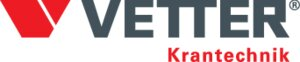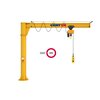Column mounted slewing jib crane for indoor use.

The Vetter PRIMUS slewing jib crane is a compact crane with infinite slewing range. It can be used in any workplace - also in small spaces and it is suitable to handle capacities up to 1.000 kg.
Jib design: Low-profile.

Advantages:

• Large effective lifting height
• Infinite slewing by 360°
• Compact design
• Easy slewing
• Small stop clearance for max utilization of effective outreach
• Can be mounted directly on a machine

Contact our crane department for more information.

• Marking: According to standard, CE-marked
• Standard: EN 13001Select a row from the table below, then click the add to quote button
I'd like to have more information
PRIMUS PR
 Outreach (m) 2 2.5 3 3.5 4 4.5 5 5.5 6 6.5 7 Capacity (kg) 30 x x x x x x x x x x x 50 x x x x x x x x x x x 80 x x x x x x x x x x x 125 x x x x x x x x x x x 200 x x x x x x x x x x x 250 x x x x x x x x x x x 400 x x x x x x x x 500 x x x x x x 800 x x x x 1000 x x x